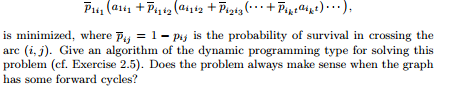### Find a forrward path whose expected length is given

Assignment Help Basic Computer Science
##### Reference no: EM131122548

(Shortest Path Problems with Losses) Consider a vehicle routing/shortest path-like problem where a vehicle wants to go on a forward path from an origin node 1 to a destination node t in a graph that has no forward cycles. For each arc (i, j) there is a given length aij , but there is also a given probability pij ∈ [0, 1] that the vehicle will be destroyed in crossing the arc. The length of a path is now a random variable, and is equal to the sum of the arc lengths on the path up to the time the vehicle reaches its destination or gets destroyed, whichever comes first. We want to find a forward path P = (1, i1,...,ik, t) whose expected length, given by#### Show that there exists at least one node j

Show that there exists at least one node j such that the sequence of labels dj generated by the algorithm diverge to -∞. Hint: Argue that if the limits dj of all the label n

#### Derive a dynamic programming algorithm that proceeds forward

Apply this transformation to the dynamic programming problem of Example 2.2 and Exercise 2.23, and derive a dynamic programming algorithm that proceeds forwards rather than

#### Denote the shortest distance from node 1 to node i

Sequentially, for k = 2, 3,..., denote by di(k) the minimum of the lengths of paths from 1 to i that have length greater than di(k - 1) [if there is no path from 1 to i with

#### Formulate this problem as a minimum cost flow problem

Formulate this problem as a minimum cost flow problem. (For an auction algorithm that solves this problem, see Bertsekas and Casta˜non [1993c].) Hint: Replace each node i ot

#### Finding a spanning tree with minimum sum of arc weights

Provide an O(N2), implementation of this algorithm. Hint: Together with the kth fragment Fk, maintain for each j /∈ Fk the node nk(i) ∈ Fk such that the arc connecting j and

#### Describe an algorithm of the ford-fulkerson type

If the supplies si and the arc flow bounds bij and cij are integer, your algorithm should be guaranteed to find an integer feasible solution (assuming at least one feasible

#### Consider a feasible max-flow problem

Show that if the upper flow bound of each arc is increased by α > 0, then the value of the maximum flow is increased by no more than αA, where A is the number of arcs.

#### Eliminate all forward paths from s to t

The maximum number of forward paths from s to t that do not share any nodes (other than s and t) is equal to the minimum number of nodes that when removed from the graph, el

### Write a Review